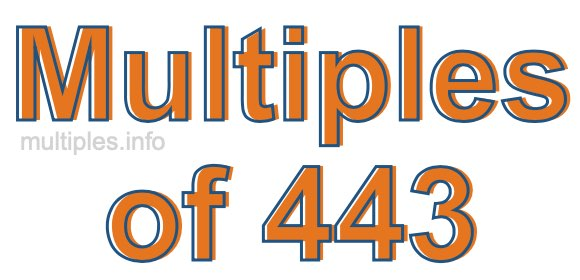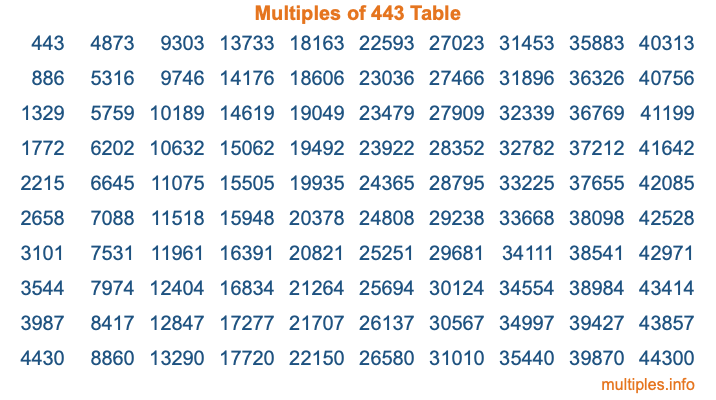Multiples of 443Welcome to the Multiples of 443 page. Here we will first teach you everything you will ever need to know about the multiples of 443, and then give you a study guide summary of everything we taught you to make sure you remember it all. Use this page to look up facts and learn information about the multiples of 443. This page will make you a multiples of four hundred forty-three expert!

Definition of Multiples of 443
Multiples of 443 are all the numbers that when divided by 443 equal an integer. Each of the multiples of 443 are called a multiple. A multiple of 443 is created by multiplying 443 by an integer.

Therefore, to create a list of multiples of 443, you start with 1 multiplied by 443, then 2 multiplied by 443, then 3 multiplied by 443, and so on for as long as you want. Thus, the list of the first five multiples of 443 is 443, 886, 1329, 1772, and 2215. To see a larger list of multiples of 443, see the printable image of Multiples of 443 further down on this page. We also have a category where you can choose any nth multiple of 443.

Multiples of 443 Checker
The Multiples of 443 Checker below checks to see if any number of your choice is a multiple of 443. In other words, it checks to see if there is any number (integer) that when multiplied by 443 will equal your number. To do that, we divide your number by 443. If the the quotient is an integer, then your number is a multiple of 443.

Is  a multiple of 443?

Least Common Multiple of 443 and ...
A Least Common Multiple (LCM) is the lowest multiple that two or more numbers have in common. This is also called the smallest common multiple or lowest common multiple and is useful to know when you are adding our subtracting fractions. Enter one or more numbers below (443 is already entered) to find the LCM.

Check out our LCM Calculator if you need more details about the Least Common Multiple or if you need the LCM for different numbers for adding and subtraction fractions.

nth Multiple of 443
As we stated above, 443 is the first multiple of 443, 886 is the second multiple of 443, 1329 is the third multiple of 443, and so on. Enter a number below to find the nth multiple of 443.

th multiple of 443

Multiples of 443 vs Factors of 443
443 is a multiple of 443 and a factor of 443, but that is where the similarities end. All postive multiples of 443 are 443 or greater than 443. All positive factors of 443 are 443 or less than 443.

Below is the beginning list of multiples of 443 and the factors of 443 so you can compare:

Multiples of 443: 443, 886, 1329, 1772, 2215, etc.

Factors of 443: 1, 443

As you can see, the multiples of 443 are all the numbers that you can divide by 443 to get a whole number. The factors of 443, on the other hand, are all the whole numbers that you can multiply by another whole number to get 443.

It's also interesting to note that if a number (x) is a factor of 443, then 443 will also be a multiple of that number (x).

Multiples of 443 vs Divisors of 443
The divisors of 443 are all the integers that 443 can be divided by evenly. Below is a list of the divisors of 443.

Divisors of 443: 1, 443

The interesting thing to note here is that if you take any multiple of 443 and divide it by a divisor of 443, you will see that the quotient is an integer.

Multiples of 443 Table
Below is an image of the first 100 multiples of 443 in a table. The table is in chronological order, column by column. The first column has the first ten multiples of 443, the second column has the next ten multiples of 443, and so on.The Multiples of 443 Table is also referred to as the 443 Times Table or Times Table of 443. You are welcome to print out our table for your studies.

Negative Multiples of 443
Although not often discussed or needed in math, it is worth mentioning that you can make a list of negative multiples of 443 by multiplying 443 by -1, then by -2, then by -3, and so on, to get the following list of negative multiples of 443:

-443, -886, -1329, -1772, -2215, etc.

Multiples of 443 Summary
Below is a summary of important Multiples of 443 facts that we have discussed on this page. To retain the knowledge on this page, we recommend that you read through the summary and explain to yourself or a study partner why they hold true.

There are an infinite number of multiples of 443.

A multiple of 443 divided by 443 will equal a whole number.

443 divided by a factor of 443 equals a divisor of 443.

The nth multiple of 443 is n times 443.

The largest factor of 443 is equal to the first positive multiple of 443.

443 is a multiple of every factor of 443.

443 is a multiple of 443.

A multiple of 443 divided by a divisor of 443 equals an integer.

443 divided by a divisor of 443 equals a factor of 443.

Any integer times 443 will equal a multiple of 443.

Multiples of a Number
Here you can get the multiples of another number, all with the same attention to detail as we did for multiples of 443 on this page.

Multiples of
Multiples of 444
Did you find our page about multiples of four hundred forty-three educational? Do you want more knowledge? Check out the multiples of the next number on our list!